# User:IssaRice/Linear algebra/Properties of a list of vectors and their images

Let$V$ and$W$ be two finite-dimensional vector spaces, let$T : V \to W$ be a linear transformation, and let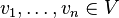$v_1, \ldots, v_n \in V$ be a list of vectors ($n$ is not necessarily equal to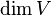$\dim V$ or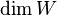$\dim W$).
Given the above background setting, we can now place various conditions on the list$v_1, \ldots, v_n$ and the transformation$T$ to conclude various things about the list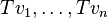$Tv_1, \ldots, Tv_n$. For instance, the first row below says that if$v_1, \ldots, v_n$ is a linearly independent list and$T$ is an injective map, then$Tv_1, \ldots, Tv_n$ is also linearly independent.
Condition on$v_1, \ldots, v_n$ Condition on$T$ Conclusion about$Tv_1, \ldots, Tv_n$ Notes
Spans$V$ Surjective Spans$W$
Basis for$V$ Bijective Basis for$W$ Combine previous two rows
Basis for$V$ No condition No conclusion Even though we have placed a strong condition on$v_1, \ldots, v_n$, if we don't place any conditions on$T$, then there is not much we can conclude about$Tv_1, \ldots, Tv_n$. If$T$ sends everything to zero, then we just get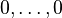$0,\ldots,0$, which neither spans$W$ nor is it a linearly independent list.
No condition Bijective No conclusion Even though we have placed a strong condition on$T$, if we don't place conditions on the list of vectors, then there is not much we can say about the list of the images. For instance, if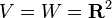$V = W = \mathbf R^2$, if$T$ is the identity map, and if our list is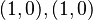$(1,0),(1,0)$, then the image is also$(1,0),(1,0)$, which is linearly dependent and does not span$W$.
Eigenvectors of$T$ corresponding to distinct non-zero eigenvalues No condition Linearly independent; eigenvectors of$T$ corresponding to distinct eigenvalues Eigenvectors corresponding to distinct eigenvalues are linearly independent, so$v_1, \ldots, v_n$ is a linearly independent list. Since each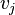$v_j$ is an eigenvector, we see that$Tv_1, \ldots, Tv_n$ is the list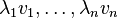$\lambda_1 v_1, \ldots, \lambda_n v_n$, where each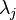$\lambda_j$ is the corresponding eigenvalue. Since by assumption none of the eigenvalues are zero, the new list is also linearly independent.